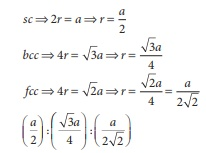# Unit VI: Solid State - Online Test

Q1. The ionic radii of A+ and B are 0.98 × 1010 m and 1.81 × 1010 m . the coordination number of each ion in AB is
Explaination / Solution:

rC+/ rA- = 0.98 ×10−10 /  1.81 ×10−10  = 0.54

it is in the range of 0. 414 - 0.732 , hence the coordination number of each ion is 6

Q2. CsCl has bcc arrangement, its unit cell edge length is 400pm, its inter atomic distance is
Explaination / Solution:

Solution

√3 a = rCs+ + 2rCl- + rCs+

(√3/2) a = ( rCl- + rCs+)

(√3/2) x 400 = inter ionic distance

Q3. A solid compound XY has NaCl structure. if the radius of the cation is 100pm , the radius of the anion will be
Explaination / Solution:

for an fcc structure rx+ / ry− = 0.414

given that rX+ = 100 pm

r y− = 100 pm  / 0.414

Q4. The vacant space in bcc lattice unit cell is
Explaination / Solution:

packing efficiency = 68%

therefore empty space percentage = (100-68) = 32%

Q5. The radius of an atom is 300pm, if it crystallizes in a face centered cubic lattice, the length oif the edge of the unit cell is
Explaination / Solution:

let edge length = a

√2a = 4r

a = [4 × 300] / √2

a = 600 ×1.414

a = 848.4 pm

Q6. The fraction of total volume occupied by the atoms in a simple cubic is
Explaination / Solution:Q7. The yellow colour in NaCl crystal is due to
Explaination / Solution:
No Explaination.

Q8. if ‘a’ stands for the edge length of the cubic system ; sc , bcc, and fcc. Then the ratio of radii of spheres in these systems will be respectively.
Explaination / Solution:Q9. If ‘a’ is the length of the side of the cube, the distance between the body centered atom and one corner atom in the cube will be
Explaination / Solution:

if a is the length of the side, then the length of the leading diagonal passing through the body centered atom is √3a

Required distance (√3/2) a

Q10. Potassium has a bcc structure with nearest neighbor distance 4.52 Aº . its atomic weight is 39. its density will be
Explaination / Solution:

ρ = n × M / a3NA

for bcc

n = 2

M=39

nearest distance 2r = 4.52